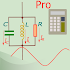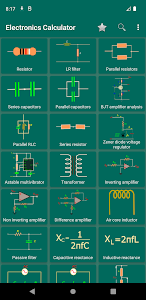# Calculatronics: EE calculators v1.18 (Paid)

### Calculatronics: EE calculators / Screenshots### Calculatronics: EE calculators / Description

Calculatronics: Electronic design calculator is an app designed for hobbyist’s DIY projects and electrical and electronics engineering/ electronics and telecommunication engineering students.

--- The app contains the following calculators ---

• 555 Timer astable multivibrator.
• 555 Timer monostable pulse duration.
• Resistor color code.
• Resistors in series calculator.
• Resistors in parallel calculator.
• Capacitors in series calculator.
• Capacitors in parallel.
• Voltage divider.
• LCR impedance calculator.
• LCR resonance calculator.
• Inverting operational amplifier (Op-amp).
• Non inverting operational amplifier (Op-amp).
• Difference operational amplifier (OP-amp).
• LED resistor value calculator.
• Zener diode voltage regulator.
• Inductor winding design calculations.
• CR passive filter calculator.
• LR passive filter Calculator .
• Capacitive Reactance.
• Inductive Reactance.
• BJT parameters analysis.
• BJT amplifier quiescent point calculator.
• Delta to star transformation calculator.
• Star to Delta transformation calculator.
• Current divider calculator.
• Parallel RLC.
• Series RLC.
• Transformer design calculator.
• Ceramic capacitor calculator.
• Polyester film capacitor calculator.
• SMD resistor calculator.
• RF calculators
• Multi layered air core inductor design calculator.
• Spiral inductor design calculator.

Calculatronics is suitable for electronics troubleshooting electronics design and electronics study

More feature will be added with each and every update. The app contains ads in order to support the development of the app.
If you have any suggestion or complaint email us please.

### Calculatronics: EE calculators / What's New in v1.18 (Paid)

- ★ Untouched Paid apk with Original Hash Signature
★ Certificate MD5 digest: 288bcfe171299f898f74f755469779bc
★ No changes were applied
➡ Languages: Full Multi Languages
➡ CPU architectures: Universal
➡ Screen DPIs: 120dpi, 160dpi, 240dpi, 320dpi, 480dpi, 640dpi

@derrin

### Choose Download Locations for Calculatronics: EE calculators v1.18 (Paid)